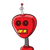# if A+B=90and tan A-3/4thenthen find the value of Cot B​

if A+B=90and tan A-3/4then
then find the value of Cot B

### 1 thought on “if A+B=90and tan A-3/4then<br />then find the value of Cot B<br />​”

1.A+B =90°

⇒A= 90°-B

tanA = 3/4

⇒tan(90°-B) = 3/4

∵ tan(90°-A) = cotA

∴ tan(90°-B) = 3/4

⇒cotB = 3/4